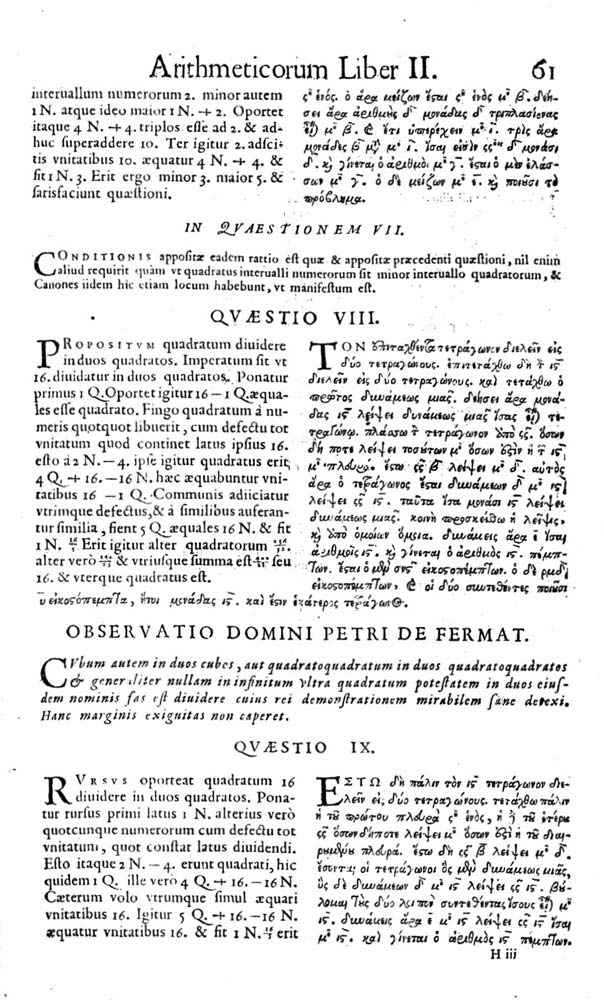﻿ Fermat's Last theoremFermat's Last theorem in commented version of Diophantus' Arithmetica

Fermat's Last theorem (Fermat's conjecture) is a renowned discrete mathematics problem introduced by amateur French mathematician of the 17th century Pierre de Fermat. Pierre de Fermat is author of many more theorems, out of which many were proved and many disproved, but it took more than 3 centuries to prove Fermat's Last theorem.

Fermat's Last theorem was published in 1670 reedition of Diophantus' Arithmetica. This edition, published by Fermat's son, was based on Bachet's latin translation of Arithmetica (1621) and comments and observations written in the page margins by Pierre de Fermat.

## Formulation

Pierre de Fermat formulated the conjecture as follows:

Cubum autem in duos cubos, aut quadratoquadratum in duos quadratoquadratos, et generaliter nullam in infinitum ultra quadratum potestatem in duos eiusdem nominis fas est dividere cuius rei demonstrationem mirabilem sane detexi. Hanc marginis exiguitas non caperet.

It is impossible to separate a cube into two cubes, or a fourth power into two fourth powers, or in general, any power higher than the second, into two like powers. I have discovered a truly marvelous proof of this, which this margin is too narrow to contain.

Written mathematically:does not hold for any## Proof after three centuries

Mathematicians tried to (dis)prove this theorem for more than three centuries. Among unsuccessful solvers famous names like EulerLaméCauchy can be found. The conjecture was proved in 1994 by English mathematician Andrew Wiles, using the means of modern mathematics of the 20th century. The proof itself has more than 100 pages and it is doubtful that some simple proof, which could Fermat had, exists.